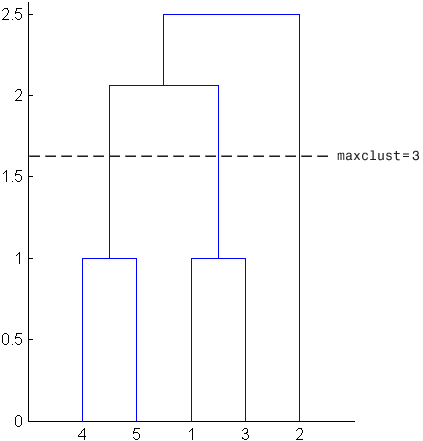## Hierarchical Clustering

### Introduction to Hierarchical Clustering

Hierarchical clustering groups data over a variety of scales by creating a cluster tree or dendrogram. The tree is not a single set of clusters, but rather a multilevel hierarchy, where clusters at one level are joined as clusters at the next level. This allows you to decide the level or scale of clustering that is most appropriate for your application. The Statistics and Machine Learning Toolbox™ function `clusterdata` supports agglomerative clustering and performs all of the necessary steps for you. It incorporates the `pdist`, `linkage`, and `cluster` functions, which you can use separately for more detailed analysis. The `dendrogram` function plots the cluster tree.

### Algorithm Description

To perform agglomerative hierarchical cluster analysis on a data set using Statistics and Machine Learning Toolbox functions, follow this procedure:

1. Find the similarity or dissimilarity between every pair of objects in the data set. In this step, you calculate the distance between objects using the `pdist` function. The `pdist` function supports many different ways to compute this measurement. See Similarity Measures for more information.

2. Group the objects into a binary, hierarchical cluster tree. In this step, you link pairs of objects that are in close proximity using the `linkage` function. The `linkage` function uses the distance information generated in step 1 to determine the proximity of objects to each other. As objects are paired into binary clusters, the newly formed clusters are grouped into larger clusters until a hierarchical tree is formed. See Linkages for more information.

3. Determine where to cut the hierarchical tree into clusters. In this step, you use the `cluster` function to prune branches off the bottom of the hierarchical tree, and assign all the objects below each cut to a single cluster. This creates a partition of the data. The `cluster` function can create these clusters by detecting natural groupings in the hierarchical tree or by cutting off the hierarchical tree at an arbitrary point.

### Note

The Statistics and Machine Learning Toolbox function `clusterdata` performs all of the necessary steps for you. You do not need to execute the `pdist`, `linkage`, or `cluster` functions separately.

### Similarity Measures

You use the `pdist` function to calculate the distance between every pair of objects in a data set. For a data set made up of m objects, there are m*(m – 1)/2 pairs in the data set. The result of this computation is commonly known as a distance or dissimilarity matrix.

There are many ways to calculate this distance information. By default, the `pdist` function calculates the Euclidean distance between objects; however, you can specify one of several other options. See `pdist` for more information.

### Note

You can optionally normalize the values in the data set before calculating the distance information. In a real world data set, variables can be measured against different scales. For example, one variable can measure Intelligence Quotient (IQ) test scores and another variable can measure head circumference. These discrepancies can distort the proximity calculations. Using the `zscore` function, you can convert all the values in the data set to use the same proportional scale. See `zscore` for more information.

For example, consider a data set, `X`, made up of five objects where each object is a set of x,y coordinates.

• Object 1: 1, 2

• Object 2: 2.5, 4.5

• Object 3: 2, 2

• Object 4: 4, 1.5

• Object 5: 4, 2.5

You can define this data set as a matrix

```rng default; % For reproducibility X = [1 2;2.5 4.5;2 2;4 1.5;... 4 2.5];```

and pass it to `pdist`. The `pdist` function calculates the distance between object 1 and object 2, object 1 and object 3, and so on until the distances between all the pairs have been calculated. The following figure plots these objects in a graph. The Euclidean distance between object 2 and object 3 is shown to illustrate one interpretation of distance.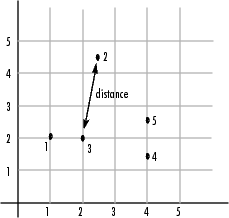#### Distance Information

The `pdist` function returns this distance information in a vector, `Y`, where each element contains the distance between a pair of objects.

`Y = pdist(X)`
```Y = 1×10 2.9155 1.0000 3.0414 3.0414 2.5495 3.3541 2.5000 2.0616 2.0616 1.0000 ```

To make it easier to see the relationship between the distance information generated by `pdist` and the objects in the original data set, you can reformat the distance vector into a matrix using the `squareform` function. In this matrix, element i,j corresponds to the distance between object i and object j in the original data set. In the following example, element 1,1 represents the distance between object 1 and itself (which is zero). Element 1,2 represents the distance between object 1 and object 2, and so on.

`squareform(Y)`
```ans = 5×5 0 2.9155 1.0000 3.0414 3.0414 2.9155 0 2.5495 3.3541 2.5000 1.0000 2.5495 0 2.0616 2.0616 3.0414 3.3541 2.0616 0 1.0000 3.0414 2.5000 2.0616 1.0000 0 ```

Once the proximity between objects in the data set has been computed, you can determine how objects in the data set should be grouped into clusters, using the `linkage` function. The `linkage` function takes the distance information generated by `pdist` and links pairs of objects that are close together into binary clusters (clusters made up of two objects). The `linkage` function then links these newly formed clusters to each other and to other objects to create bigger clusters until all the objects in the original data set are linked together in a hierarchical tree.

For example, given the distance vector `Y` generated by `pdist` from the sample data set of x- and y-coordinates, the `linkage` function generates a hierarchical cluster tree, returning the linkage information in a matrix, `Z`.

`Z = linkage(Y)`
```Z = 4×3 4.0000 5.0000 1.0000 1.0000 3.0000 1.0000 6.0000 7.0000 2.0616 2.0000 8.0000 2.5000 ```

In this output, each row identifies a link between objects or clusters. The first two columns identify the objects that have been linked. The third column contains the distance between these objects. For the sample data set of x- and y-coordinates, the `linkage` function begins by grouping objects 4 and 5, which have the closest proximity (distance value = 1.0000). The `linkage` function continues by grouping objects 1 and 3, which also have a distance value of 1.0000.

The third row indicates that the `linkage` function grouped objects 6 and 7. If the original sample data set contained only five objects, what are objects 6 and 7? Object 6 is the newly formed binary cluster created by the grouping of objects 4 and 5. When the `linkage` function groups two objects into a new cluster, it must assign the cluster a unique index value, starting with the value m + 1, where m is the number of objects in the original data set. (Values 1 through m are already used by the original data set.) Similarly, object 7 is the cluster formed by grouping objects 1 and 3.

`linkage` uses distances to determine the order in which it clusters objects. The distance vector `Y` contains the distances between the original objects 1 through 5. But linkage must also be able to determine distances involving clusters that it creates, such as objects 6 and 7. By default, `linkage` uses a method known as single linkage. However, there are a number of different methods available. See the `linkage` reference page for more information.

As the final cluster, the `linkage` function grouped object 8, the newly formed cluster made up of objects 6 and 7, with object 2 from the original data set. The following figure graphically illustrates the way `linkage` groups the objects into a hierarchy of clusters.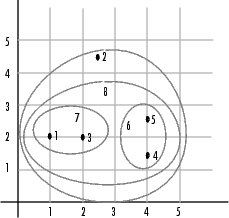### Dendrograms

The hierarchical, binary cluster tree created by the `linkage` function is most easily understood when viewed graphically. The Statistics and Machine Learning Toolbox function `dendrogram` plots the tree as follows.

`dendrogram(Z)`In the figure, the numbers along the horizontal axis represent the indices of the objects in the original data set. The links between objects are represented as upside-down U-shaped lines. The height of the U indicates the distance between the objects. For example, the link representing the cluster containing objects 1 and 3 has a height of 1. The link representing the cluster that groups object 2 together with objects 1, 3, 4, and 5, (which are already clustered as object 8) has a height of 2.5. The height represents the distance `linkage` computes between objects 2 and 8. For more information about creating a dendrogram diagram, see the `dendrogram` reference page.

### Verify the Cluster Tree

After linking the objects in a data set into a hierarchical cluster tree, you might want to verify that the distances (that is, heights) in the tree reflect the original distances accurately. In addition, you might want to investigate natural divisions that exist among links between objects. Statistics and Machine Learning Toolbox functions are available for both of these tasks, as described in the following sections.

#### Verify Dissimilarity

In a hierarchical cluster tree, any two objects in the original data set are eventually linked together at some level. The height of the link represents the distance between the two clusters that contain those two objects. This height is known as the cophenetic distance between the two objects. One way to measure how well the cluster tree generated by the `linkage` function reflects your data is to compare the cophenetic distances with the original distance data generated by the `pdist` function. If the clustering is valid, the linking of objects in the cluster tree should have a strong correlation with the distances between objects in the distance vector. The `cophenet` function compares these two sets of values and computes their correlation, returning a value called the cophenetic correlation coefficient. The closer the value of the cophenetic correlation coefficient is to 1, the more accurately the clustering solution reflects your data.

You can use the cophenetic correlation coefficient to compare the results of clustering the same data set using different distance calculation methods or clustering algorithms. For example, you can use the `cophenet` function to evaluate the clusters created for the sample data set.

`c = cophenet(Z,Y)`
```c = 0.8615 ```

`Z` is the matrix output by the `linkage` function and `Y` is the distance vector output by the `pdist` function.

Execute `pdist` again on the same data set, this time specifying the city block metric. After running the `linkage` function on this new `pdist` output using the average linkage method, call `cophenet` to evaluate the clustering solution.

```Y = pdist(X,'cityblock'); Z = linkage(Y,'average'); c = cophenet(Z,Y)```
```c = 0.9047 ```

The cophenetic correlation coefficient shows that using a different distance and linkage method creates a tree that represents the original distances slightly better.

#### Verify Consistency

One way to determine the natural cluster divisions in a data set is to compare the height of each link in a cluster tree with the heights of neighboring links below it in the tree.

A link that is approximately the same height as the links below it indicates that there are no distinct divisions between the objects joined at this level of the hierarchy. These links are said to exhibit a high level of consistency, because the distance between the objects being joined is approximately the same as the distances between the objects they contain.

On the other hand, a link whose height differs noticeably from the height of the links below it indicates that the objects joined at this level in the cluster tree are much farther apart from each other than their components were when they were joined. This link is said to be inconsistent with the links below it.

In cluster analysis, inconsistent links can indicate the border of a natural division in a data set. The `cluster` function uses a quantitative measure of inconsistency to determine where to partition your data set into clusters.

The following dendrogram illustrates inconsistent links. Note how the objects in the dendrogram fall into two groups that are connected by links at a much higher level in the tree. These links are inconsistent when compared with the links below them in the hierarchy.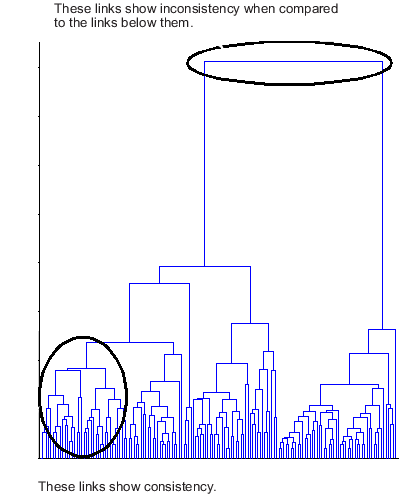The relative consistency of each link in a hierarchical cluster tree can be quantified and expressed as the inconsistency coefficient. This value compares the height of a link in a cluster hierarchy with the average height of links below it. Links that join distinct clusters have a high inconsistency coefficient; links that join indistinct clusters have a low inconsistency coefficient.

To generate a listing of the inconsistency coefficient for each link in the cluster tree, use the `inconsistent` function. By default, the `inconsistent` function compares each link in the cluster hierarchy with adjacent links that are less than two levels below it in the cluster hierarchy. This is called the depth of the comparison. You can also specify other depths. The objects at the bottom of the cluster tree, called leaf nodes, that have no further objects below them, have an inconsistency coefficient of zero. Clusters that join two leaves also have a zero inconsistency coefficient.

For example, you can use the `inconsistent` function to calculate the inconsistency values for the links created by the `linkage` function in Linkages.

First, recompute the distance and linkage values using the default settings.

```Y = pdist(X); Z = linkage(Y);```

Next, use `inconsistent` to calculate the inconsistency values.

`I = inconsistent(Z)`
```I = 4×4 1.0000 0 1.0000 0 1.0000 0 1.0000 0 1.3539 0.6129 3.0000 1.1547 2.2808 0.3100 2.0000 0.7071 ```

The `inconsistent` function returns data about the links in an (m-1)-by-4 matrix, whose columns are described in the following table.

ColumnDescription

1

Mean of the heights of all the links included in the calculation

2

Standard deviation of all the links included in the calculation

3

Number of links included in the calculation

4

Inconsistency coefficient

In the sample output, the first row represents the link between objects 4 and 5. This cluster is assigned the index 6 by the `linkage` function. Because both 4 and 5 are leaf nodes, the inconsistency coefficient for the cluster is zero. The second row represents the link between objects 1 and 3, both of which are also leaf nodes. This cluster is assigned the index 7 by the linkage function.

The third row evaluates the link that connects these two clusters, objects 6 and 7. (This new cluster is assigned index 8 in the `linkage` output). Column 3 indicates that three links are considered in the calculation: the link itself and the two links directly below it in the hierarchy. Column 1 represents the mean of the heights of these links. The `inconsistent` function uses the height information output by the `linkage` function to calculate the mean. Column 2 represents the standard deviation between the links. The last column contains the inconsistency value for these links, 1.1547. It is the difference between the current link height and the mean, normalized by the standard deviation.

`(2.0616 - 1.3539) / .6129`
```ans = 1.1547 ```

The following figure illustrates the links and heights included in this calculation.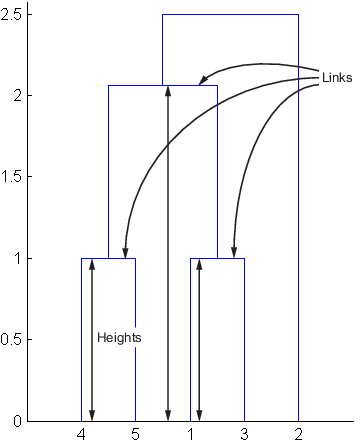### Note

In the preceding figure, the lower limit on the y-axis is set to `0` to show the heights of the links. To set the lower limit to `0`, select ```Axes Properties``` from the Edit menu, click the tab, and enter `0` in the field immediately to the right of Y Limits.

Row 4 in the output matrix describes the link between object 8 and object 2. Column 3 indicates that two links are included in this calculation: the link itself and the link directly below it in the hierarchy. The inconsistency coefficient for this link is 0.7071.

The following figure illustrates the links and heights included in this calculation.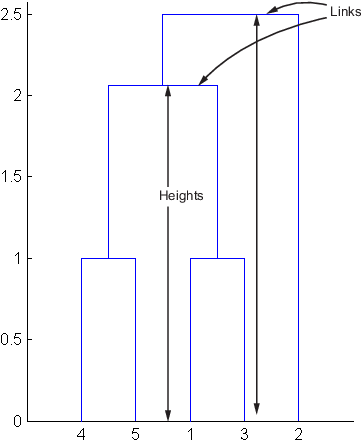### Create Clusters

After you create the hierarchical tree of binary clusters, you can prune the tree to partition your data into clusters using the `cluster` function. The `cluster` function lets you create clusters in two ways, as discussed in the following sections:

#### Find Natural Divisions in Data

The hierarchical cluster tree may naturally divide the data into distinct, well-separated clusters. This can be particularly evident in a dendrogram diagram created from data where groups of objects are densely packed in certain areas and not in others. The inconsistency coefficient of the links in the cluster tree can identify these divisions where the similarities between objects change abruptly. (See Verify the Cluster Tree for more information about the inconsistency coefficient.) You can use this value to determine where the `cluster` function creates cluster boundaries.

For example, if you use the `cluster` function to group the sample data set into clusters, specifying an inconsistency coefficient threshold of `1.2` as the value of the `cutoff` argument, the `cluster` function groups all the objects in the sample data set into one cluster. In this case, none of the links in the cluster hierarchy had an inconsistency coefficient greater than `1.2`.

`T = cluster(Z,'cutoff',1.2)`
```T = 5×1 1 1 1 1 1 ```

The `cluster` function outputs a vector, `T`, that is the same size as the original data set. Each element in this vector contains the number of the cluster into which the corresponding object from the original data set was placed.

If you lower the inconsistency coefficient threshold to `0.8`, the `cluster` function divides the sample data set into three separate clusters.

`T = cluster(Z,'cutoff',0.8)`
```T = 5×1 3 2 3 1 1 ```

This output indicates that objects 1 and 3 are in one cluster, objects 4 and 5 are in another cluster, and object 2 is in its own cluster.

When clusters are formed in this way, the cutoff value is applied to the inconsistency coefficient. These clusters may, but do not necessarily, correspond to a horizontal slice across the dendrogram at a certain height. If you want clusters corresponding to a horizontal slice of the dendrogram, you can either use the `criterion` option to specify that the cutoff should be based on distance rather than inconsistency, or you can specify the number of clusters directly as described in the following section.

#### Specify Arbitrary Clusters

Instead of letting the `cluster` function create clusters determined by the natural divisions in the data set, you can specify the number of clusters you want created.

For example, you can specify that you want the `cluster` function to partition the sample data set into two clusters. In this case, the `cluster` function creates one cluster containing objects 1, 3, 4, and 5 and another cluster containing object 2.

`T = cluster(Z,'maxclust',2)`
```T = 5×1 2 1 2 2 2 ```

To help you visualize how the `cluster` function determines these clusters, the following figure shows the dendrogram of the hierarchical cluster tree. The horizontal dashed line intersects two lines of the dendrogram, corresponding to setting `'maxclust'` to `2`. These two lines partition the objects into two clusters: the objects below the left-hand line, namely 1, 3, 4, and 5, belong to one cluster, while the object below the right-hand line, namely 2, belongs to the other cluster.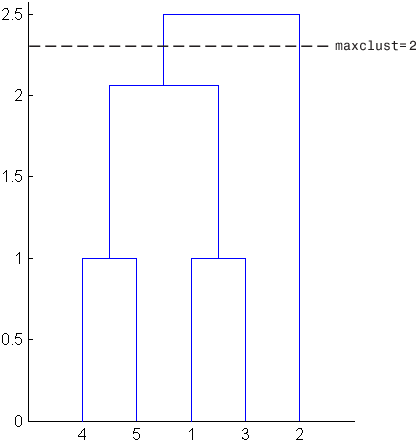On the other hand, if you set `'maxclust'` to  `3`, the cluster function groups objects 4 and 5 in one cluster, objects 1 and 3 in a second cluster, and object 2 in a third cluster. The following command illustrates this.

`T = cluster(Z,'maxclust',3)`
```T = 5×1 2 3 2 1 1 ```

This time, the `cluster` function cuts off the hierarchy at a lower point, corresponding to the horizontal line that intersects three lines of the dendrogram in the following figure.